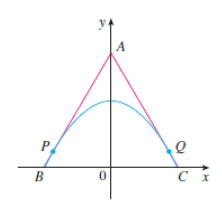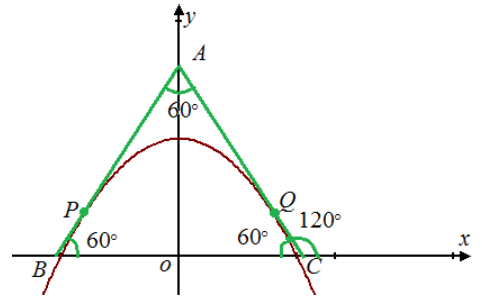# The point P and Q on the parabola y = 1 − x 2 .### Single Variable Calculus: Concepts...

4th Edition
James Stewart
Publisher: Cengage Learning
ISBN: 9781337687805### Single Variable Calculus: Concepts...

4th Edition
James Stewart
Publisher: Cengage Learning
ISBN: 9781337687805

#### Solutions

Chapter 2, Problem 11P
To determine

## To find:The point P and Q on the parabola y=1−x2 .

Expert Solution

The value of the points P and Q on the parabola y=1x2 are P=(32,14),Q=(32,14) .

### Explanation of Solution

Given information:

The given equation for parabola is y=1x2 .

The given equilateral triangle is shown in figure (1).Figure (1)

Calculation:

The parabola is y=1x2 .

The triangle ABC is an equilateral shown in figure (2).Figure (2)

Each angle in triangle ABC is equal to 60° , the slope of the tangent AB which makes an angle of 60° with positive x-axis.

m1=tan60°=3

The slope of the AC which makes an angle of 120° with positive x-axis.

m2=tan120°=tan(180°60°)=tan60°=3

The slope of the tangent to the parabola y=1x2 at P , Q are as.

y'=2x

Substitute 3 for m1 and, 3 for m2 in above equation.

±3=2xx=±32

Calculate the values of P , Q points on parabola.

Substitute ±32 for x in equation y=1x2 .

y=1(±32)2=134=14

The points P and Q are P=(32,14),Q=(32,14)

Therefore, the value of the points P and Q on the parabola y=1x2 are P=(32,14),Q=(32,14) .

### Have a homework question?

Subscribe to bartleby learn! Ask subject matter experts 30 homework questions each month. Plus, you’ll have access to millions of step-by-step textbook answers!